# KSEEB Solutions for Class 9 Maths Chapter 3 Lines and Angles Ex 3.1

KSEEB Solutions for Class 9 Maths Chapter 3 Lines and Angles Ex 3.1 are part of KSEEB Solutions for Class 9 Maths. Here we have given Karnataka Board Class 9 Maths Chapter 3 Lines and Angles Exercise 3.1.

## Karnataka Board Class 9 Maths Chapter 3 Lines and Angles Ex 3.1

KSEEB Solutions For Class 9 Maths Lines And Angles Question 1.
In Fig 3.13, lines AB and CD intersect at O. If ∠AOC + ∠BOE = 70° and ∠BOD = 40°, find ∠BOE and reflex ∠COE.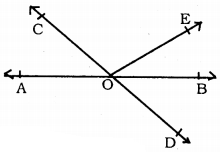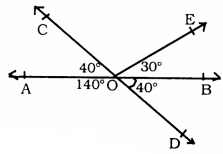∠AOC = ∠BOD = 40°
∠BOD = 40° (Date)
∠AOC = ∠BOD = 40° because vertifically opposite angles.
∴ ∠AOC + ∠BOE = 70°
40° + ∠BOE = 70°
∴ ∠BOE = 70° – 40° = 30°, and
∠AOD = 180° – ∠BOD = 180° – 40° = 140°
Reflex angle COE = ∠COA + ∠AOD + ∠BOD + ∠BOE
= 40° + 140° + 40° + 30°
∴ Reflex angle, ∠COE = 250°.

Lines And Angles Class 9 Exercise 3.1 Question 2.
In Fig. 3.14, lines XY and MN intersect at O. If ∠POY = 90° and a : b = 2 : 3, find c.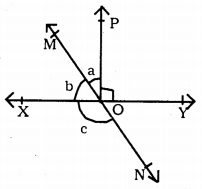∠XOP + ∠POY = 180°
∵ straight supplementary
∠XOP + 90° =180°
∴ ∠XOP = 180° – 90° = 90°
But, ∠XOP = a + b = 90°
a : b = 2 : 3
2 + 3 = 5 Ratio
Ratio 5 means 90°
$$=\frac{2 \times 90}{5}=2 \times 18=36^{\circ}$$
∴ If a = 36° then, ∠b = 54°.
∠XOM = ∠YON = b = 54° (∵ Vertically opposite angles)
∠XON + ∠YON = 180° (∵ Straight angle)
∴ c + 54° = 180°
c = 180 – 54
∴ c = 126°.

KSEEB Solutions For Class 9 Maths Question 3.
In Fig. 3.15, ∠PQR = ∠PRQ, then prove that ∠PQS = ∠PRT.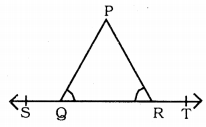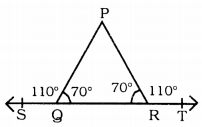Data: In this figure, ∠PQR = ∠PRQ.
To prove: ∠PQS = ∠PRT
Proof: In ∆PQR, ∠Q = ∠R.
∴ This is an isosceles triangle.
Let ∠Q = 70°, then ∠R = 70° .
∠PQS + ∠PQR = 180°
∠PQS + 70° = 180°
∴ ∠PQS =180 – 70
∠PQS = 110° … (i)
Similarly,
∠PRT + ∠PRQ = 180°
∠PRT + 70° = 180°
∴ ∠PRT = 180 – 70
∠PRT =100 … (ii)
from (i) and (ii)
∠PQS = ∠PRT =110°
∴ ∠PQS = ∠PRS proved.

KSEEB Solutions For Class 9 Maths Chapter 3 Question 4.
If Fig. 3.16, if x + y = w + z, then prove that AOB is a line.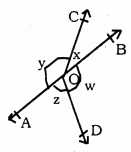Data: In this fiure, ∠BOC = x°
∠AOC = y°
∠BOD = w°
∠AOD = z° and
x + y = w + z.
To Prove: AOB is a straight line.
Proof: x + y + w + z = 360° (∵ one completre angle)
But, x + y = w + z.
∴ x + y = w + z= $$\frac{360}{2}$$ = 180°
∴ x + y = 180°
∴ w + z = 180° proved.
But, ∠AOC and ∠BOC are adjacent angles,
∠AOC + ∠BOC = 180°
x = y = 180°
∴ ∠AOB = 180°.
∴ AOB is a straight line.

Lines And Angles Class 9 KSEEB Question 5.
In Fig. 3.17, POQ is a line. Ray OR is perpendicular to line PQ. OS is another ray lying between rays OP and OR. Prove that
∠ROS = $$\frac{1}{2}$$ (∠QOS – ∠POS).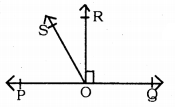Data: POQ is a straight line. Ray OR is perpendicular on straight line PQ. OS Ray is in between Rays OP and OR.
To Prove: ∠ROS = $$\frac{1}{2}$$ (∠QOS – ∠POS)
Proof : ∠QOR = ∠POR = 90° (Data)
∠POR = ∠POS + ∠ROS
∴ ∠ROS = ∠POR – ∠POS
∠ROS = 90°- ∠POS (i)
Now, QOS = ∠QOR + ∠ROS
∠QOS = 90° + ∠ROS
∴ ∠ROS = ∠QOS – 90° (ii)
By adding equation (i) and (ii)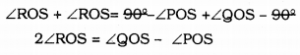∴ ∠ROS = $$\frac{1}{2}$$ (∠QOS – ∠POS)

Lines And Angles Exercise 3.1 Question 6.
It is given that ∠XYZ = 64° and XY is produced to point P. Draw a figure from the given information. If ray YQ bisects ∠ZYP, find ∠XYO and reflex ∠QYP.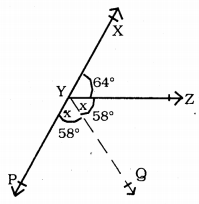Data: ∠XYZ = 64° and XY are produced up to P. ∠ZYP is bisected.
To Prove: ∠XYQ = ? Reflex ∠QYP = ?
Proof: YQ bisects ∠ZYP
∴ Let ∠PYQ = ∠ZYQ = x°.
∠XYZ + ∠ZYQ + ∠QYP = 180° (∵ ∠XYP is straight angle)
64 + x + x = 180
∴ 64 + 2x = 180
∴ 2x = 180 – 64
2x = 116
∴ $$x=\frac{116}{2}=58^{\circ}$$
∴ ∠PYQ = ∠ZYQ = 58°

(i) ∴ ∠XYQ = ∠XYZ + ∠ZYQ
= 64 + 58
∠XYQ = 122°

(ii) Reflex ∠QYP = ∠PYX + ∠XYZ + ∠ZYQ
= 180 ° + 64° + 58°
= 180 + 122
∴ Reflex ∠QYP = 302°.

We hope the KSEEB Solutions for Class 9 Maths Chapter 3 Lines and Angles Ex 3.1 help you. If you have any query regarding Karnataka Board Class 9 Maths Chapter 3 Lines and Angles Exercise 3.1, drop a comment below and we will get back to you at the earliest.